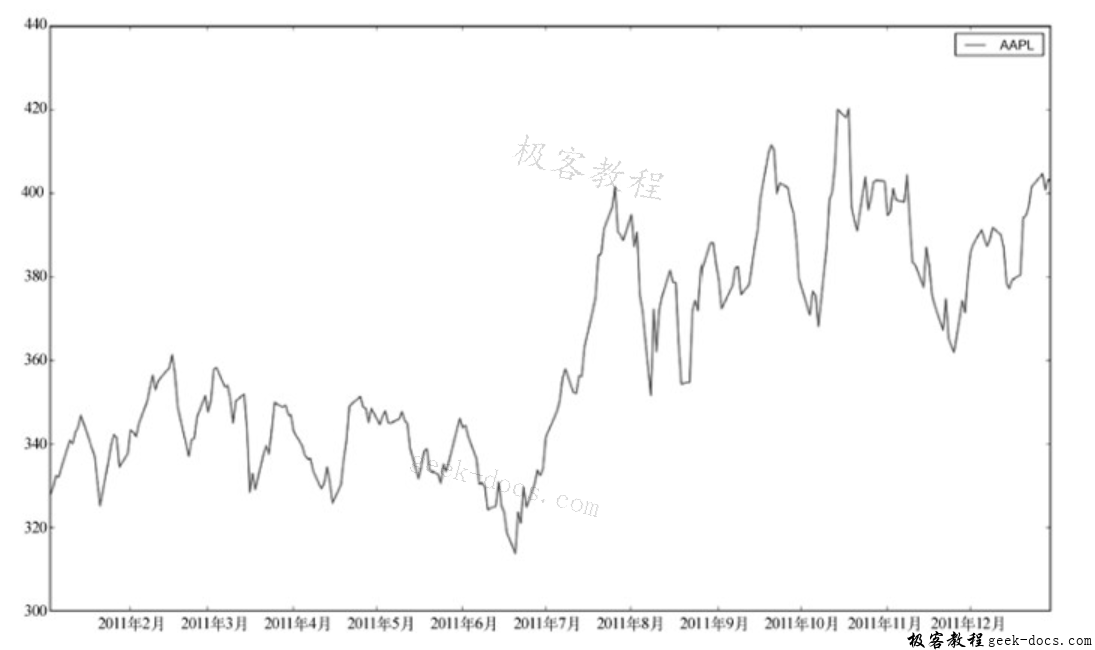# Numpy 重采样时间序列数据

## 具体步骤

1. 创建一个日期时间索引对象。

dt_idx = pandas.DatetimeIndex(quotes.date)


1. 创建DataFrame对象。

df = pandas.DataFrame(quotes.close, index=dt_idx,
columns=[symbol])


1. 重采样。

resampled = df.resample('M', how=numpy.mean)
print resampled



AAPL
2011-01-31 336.932500
2011-02-28 349.680526
2011-03-31 346.005652
2011-04-30 338.960000
2011-05-31 340.324286
2011-06-30 329.664545
2011-07-31 370.647000
2011-08-31 375.151304
2011-09-30 390.816190
2011-10-31 395.532381
2011-11-30 383.170476
2011-12-31 391.251429


1. 绘图。

DataFrameplot方法，绘制数据。

df.plot()
resampled.plot()
show()import pandas
from matplotlib.pyplot import show, legend
from datetime import datetime
from matplotlib import finance
import numpy

# 下载2011年到2012年的AAPL股价数据
start = datetime(2011, 01, 01)
end = datetime(2012, 01, 01)

symbol = "AAPL"
quotes = finance.quotes_historical_yahoo(symbol, start, end, asobject=True)

# 创建日期时间索引对象
dt_idx = pandas.DatetimeIndex(quotes.date)

# 创建DataFrame对象
df = pandas.DataFrame(quotes.close, index=dt_idx, columns=[symbol])

# 以每月一次的频率重采样
resampled = df.resample('M', how=numpy.mean)
print resampled

# 绘图
df.plot()
resampled.plot()
show()



## 攻略小结

• D，每天一次
• M，每月一次
• A，每年一次

resample方法中的how参数指明了具体的重采样方式，默认的重采样方式是计算平均值。

• 回顶